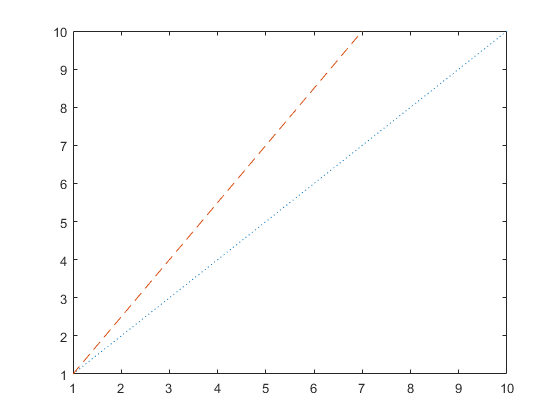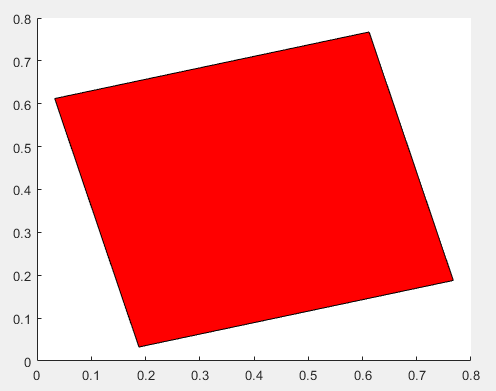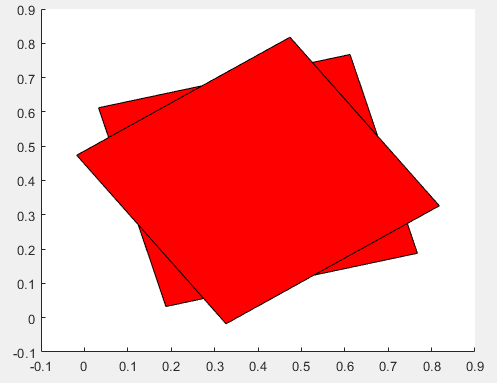# arguments

## 语法

```arguments
`argName1 (dimensions) class {validators} = defaultValue`
...
`argNameN ...`
end

arguments (Repeating)
`argName1 (dimensions) class {validators} = defaultValue`
...
`argNameN ...`
end

arguments (Output)
`argName1 (dimensions) class {validators}`
...
`argNameN ...`
end

arguments (Output,Repeating)
`argName (dimensions) class {validators}`
end

```

## 说明

### 输入参数块

````arguments ... end` 声明函数的输入参数。参数块是可选的。如果包含一个或多个 `arguments` 块，它们必须出现在函数的第一个可执行代码行之前。MATLAB® 将任何未显式标记为 `Input` 或 `Output` 的参数块视为输入块。每个参数可以有一个或多个约束或一个默认值，如以下语法所示：`argName (dimensions) class {validators} = defaultValue` `(dimensions)` - 输入大小，指定为包含两个或多个数值的以逗号分隔的列表，如 `(1,2)`、`(3,5,2)` 或 `(1,:)`。冒号表示该维度可以包含任意长度。`(dimensions)` 不能包含表达式。输入的维度必须与 `(dimensions)` 完全匹配，或与 `(dimensions)` 指定的大小兼容。例如，`(1,:)` 指定输入必须为 1×n 行向量，但 n×1 列向量是兼容的。该函数将行向量输入重构为列向量。同样，大小为 `(2,3)` 的值允许标量输入，但它将输入扩展为 2×3 矩阵。有关详细信息，请参阅 基本运算的兼容数组大小。`class` - 按名称指定的类或 MATLAB 数据类型，如 `double`。输入必须为指定的类型或可以转换为该类型的类型。例如，指定 `double` 的函数接受 `single` 类的值并将它们转换为 `double`。有关转换的详细信息，请参阅Implicit Class Conversion。`{validators}` - 以逗号分隔的验证函数（如 `mustBeNumeric` 和 `mustBeScalarOrEmpty`）列表，用花括号括起来。当输入参数与其条件不匹配时，验证函数会出错。与 `class` 不同，验证函数不修改输入参数。有关验证函数的列表，请参阅 参数验证函数。`defaultValue` - 默认值必须符合指定的大小、类型和验证规则。默认值也可以是表达式。指定默认值会使该参数成为可选参数。可选参数必须位于函数签名中和 `arguments` 块中的必需参数后。 对于名称-值参数，`arg` 使用 `nv.name` 的形式，其中 `nv` 是函数签名中的结构体名称，`name` 是参数块中的参数名称。例如，使用名为 `options` 的结构体定义接受名称-值参数的函数。 y = myFunction(x,options) 在参数块中，将名称-值参数的名称指定为字段：arguments x options.Name1 options.Name2 end 有关通常情况下使用 `arguments` 块的详细信息，请参阅 arguments 代码块语法。```

``arguments (Repeating) ... end` 声明重复输入参数。例如，如果创建一个名为 `myplot` 且包含重复参数 `X`、`Y` 和 `style` 的函数，则该函数接受这三个参数的多个组，例如 `myplot(x1,y1,style1,x2,y2,style2)`。MATLAB 创建一个元胞数组，其中包含为该参数传入的所有值。 函数只能包含一个重复输入参数块。如果该函数同时包含重复参数和名称-值参数，请在重复参数块后在单独的参数块中声明名称-值参数。有关对重复参数使用验证的详细信息，请参阅验证重复参数。`

### 输出参数块

``arguments (Output) ... end` 声明函数的输出参数。输出参数块是可选的。如果包含一个或多个输出 `arguments` 块，它们必须出现在所有输入块之后，函数的第一个可执行代码行之前。当在函数中同时包含输入和输出块时，为了提高可读性，建议显式包含 `(Input)` 和 `(Output)` 属性。请参阅使用参数验证重复输出中的示例。（从 R2022b 开始）与输入参数一样，输出参数也可以有一个或多个约束，如以下语法所示：`argName (dimensions) class {validators}`有关其他详细信息，请参阅 `arguments ... end` 的描述。与输入参数不同，输出参数无法定义默认值，应用于输出参数的验证函数无法引用早期的输出参数。`

` `arguments (Output,Repeating) ... end` 为函数声明重复输出参数。可以对重复输出参数使用参数验证，但每个函数只能定义一个重复输出参数。`varargout` 可以出现在重复输出参数块中，只要它是唯一的输出参数即可。（从 R2022b 开始）`

## 示例

```function [m,s] = twoStats(x) arguments x (1,:) {mustBeNumeric} end m = mean(x,"all"); s = std(x,1,"all"); end```

```a = [1 3 5]; [m,s] = twoStats(a)```
```m = 3 s = 1.6330```

```a = [1 3 5]'; [m,s] = twoStats(a)```
```m = 3 s = 1.6330```

```a = ["1" "3" "5"]; [m,s] = twoStats(a)```
```Error using twoStats Invalid argument at position 1. Value must be numeric.```

```function myRectangle(X,Y,options) arguments X double Y double options.LineStyle (1,1) string = "-" options.LineWidth (1,1) {mustBeNumeric} = 1 end % Function code ... end ```

```myRectangle(4,5) myRectangle(4,5,LineStyle=":",LineWidth=2) myRectangle(4,5,LineWidth=2,LineStyle=":") myRectangle(4,5,LineStyle=":") myRectangle(4,5,LineWidth=2) ```

```function fRepeat(x,y,style) arguments (Repeating) x (1,:) double y (1,:) double style {mustBeMember(style,["--",":"])} end % Reshape the cell arrays of inputs and call plot function z = reshape([x;y;style],1,[]); if ~isempty(z) plot(z{:}); end end```

```x1 = 1:10; y1 = 1:10; s1 = ":"; x2 = 1:7; y2 = 1:1.5:10; s2 = "--"; fRepeat(x1,y1,s1,x2,y2,s2) ``````function [xfinal,yfinal] = rotatePatch(angle) arguments (Output) xfinal {mustBePositive} yfinal {mustBePositive} end x = [0.1 0.1 0.7 0.7]; y = [0.1 0.7 0.7 0.1]; p = patch(x,y,"red"); rotate(p,[0 0 1],angle,[0.4 0.4 0]) xfinal = p.Vertices(:,1); yfinal = p.Vertices(:,2); end```

`[x1,y1] = rotatePatch(15)`
```x1 = 0.1879 0.0326 0.6121 0.7674 y1 = 0.0326 0.6121 0.7674 0.1879````[x2,y2] = rotatePatch(35)`
```Invalid output 'xfinal'. Value must be positive. Error in rotatePatch (line 12) end``````function vectorSum = repeatSum(a,b) arguments (Input,Repeating) a (1,:) b (1,:) end arguments (Output,Repeating) vectorSum (1,:) end n = numel(a); vectorSum{n} = a{n} + b{n}; for i = 1:n-1 vectorSum{i} = a{i} + b{i}; end end ```

`for` 循环为元胞数组预分配空间之前，计算最终输出并将其赋给 `vectorSum{n}`。在没有预分配空间的情况下在循环中扩展元胞数组有可能会对性能产生负面影响。

```x1 = [1 2]; y1 = [3 4]; x2 = [1; 0]; y2 = [0; 1]; [sum1,sum2] = repeatSum(x1,y1,x2,y2)```
```sum1 = 4 6 sum2 = 1 1```

`x1 + y2`
```ans = 1 2 2 3 ```

## 局限性

• 嵌套函数、抽象方法或句柄类析构函数方法不支持参数块。

## 提示

• 使用数据类型限制会导致输入参数的隐式转换。例如：

```function y = myFunction(inputArg1) arguments inputArg1 (1,1) double end ...```
对于此函数，如果将字符串 `"123"` 作为输入参数传递，则 MATLAB 会将字符串转换为 `double` 类型的数值 `123`

验证函数不会以任何方式更改输入值，因此为了避免数据类型转换，请使用一个或多个验证器函数而不是数据类型来限制输入。例如：

• 要避免将字符串转换为数值，请使用 `mustBeA``mustBeFloat``mustBeNumeric`

• 要避免将数值转换为字符串，请使用 `mustBeText``mustBeTextScalar``mustBeNonZeroLengthText`

• 要避免大小转换，请使用 `mustBeVector``mustBeScalarOrEmpty`